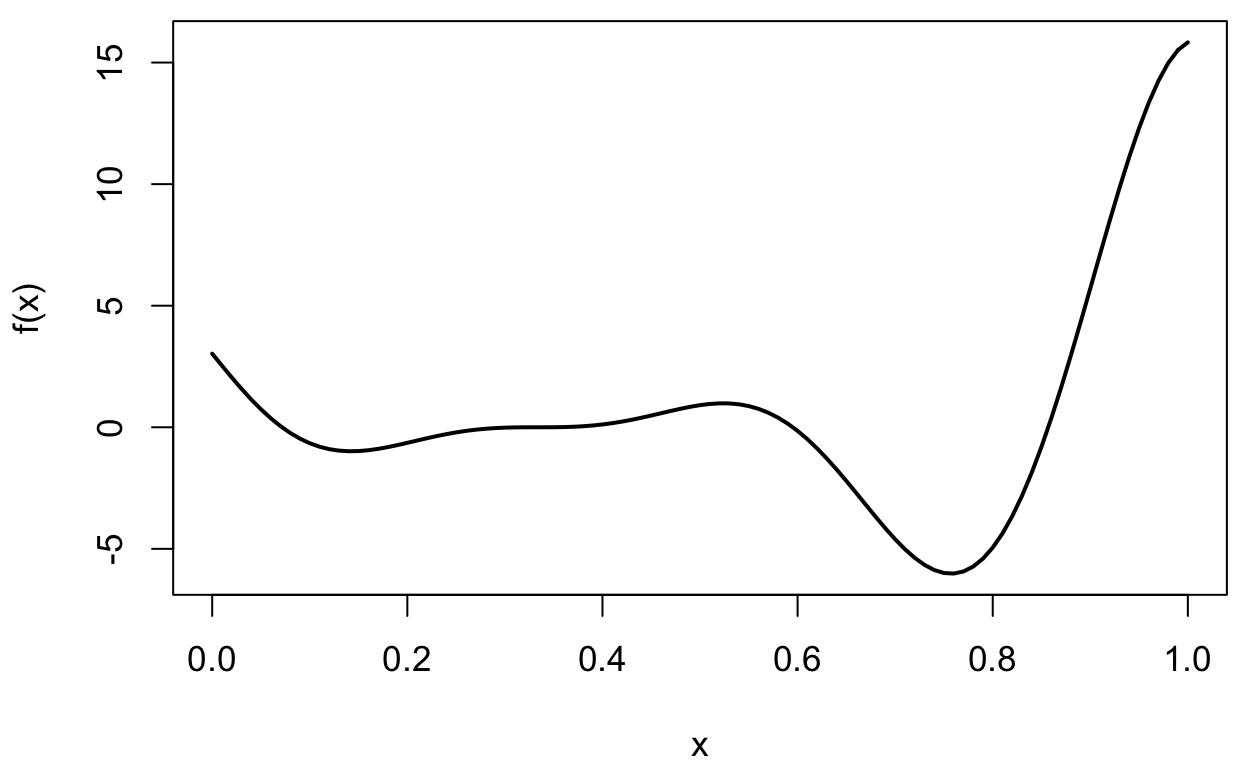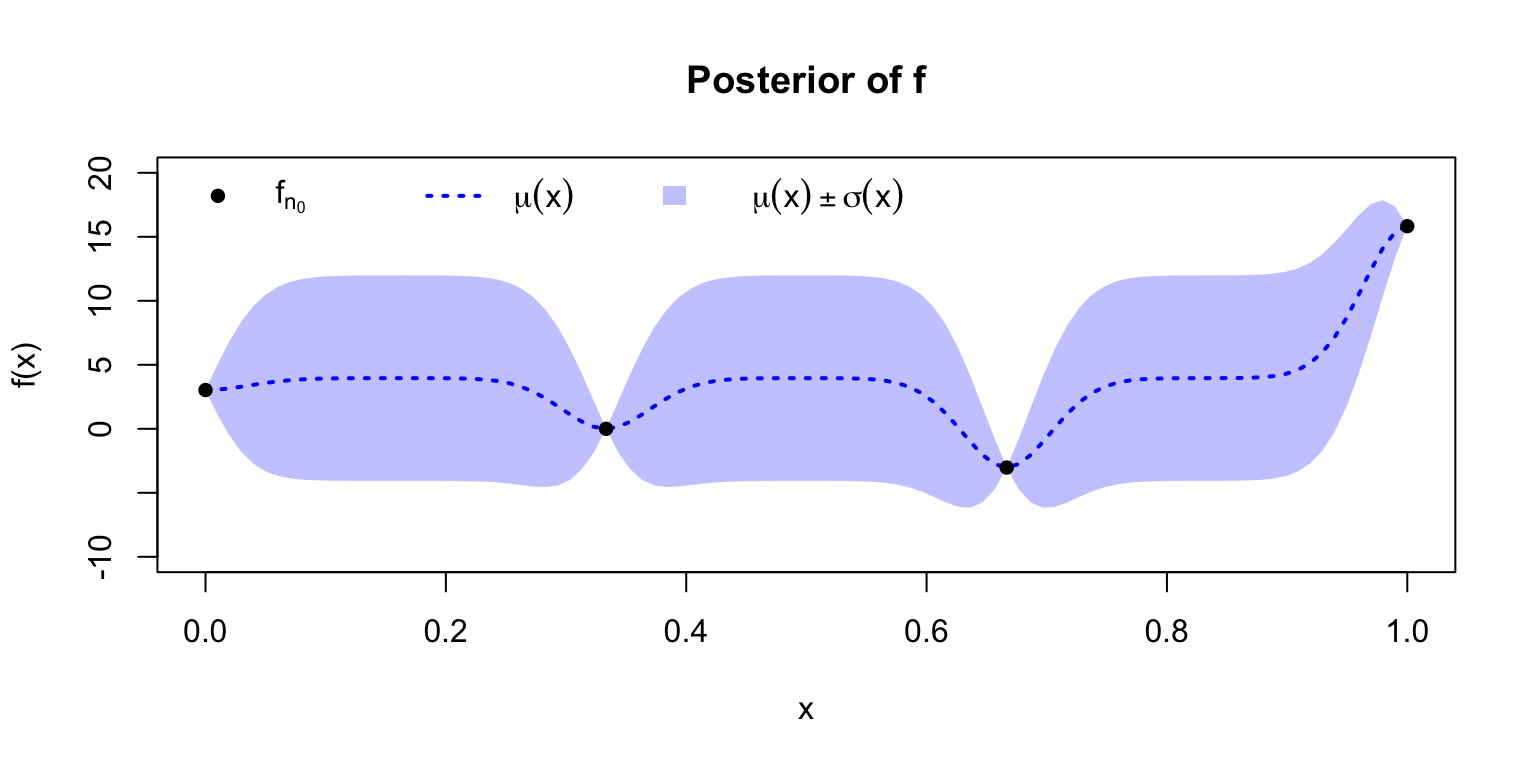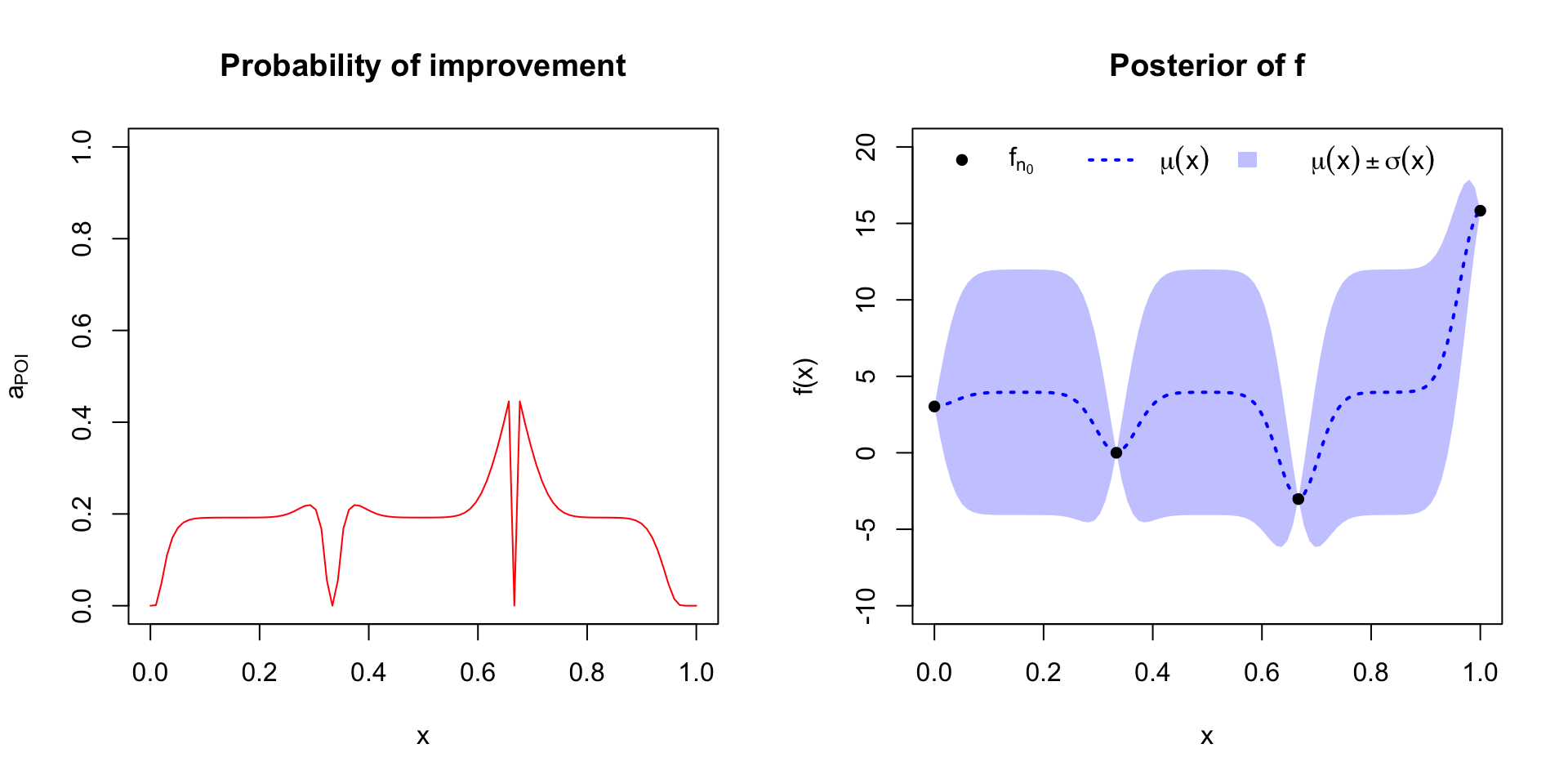# A tutorial on Bayesian optimization in R

Step-by-step demonstration of BayesOpt for derivative-free minimization of a noiseless, black-box function

Mikhail Popov https://mpopov.com
2019-05-19

# Introduction

Optimization of function $$f$$ is finding an input value $$\mathbf{x}_*$$ which minimizes (or maximizes) the output value:

$\mathbf{x}_* = \underset{\mathbf{x}}{\arg\min}~f(\mathbf{x})$

In this tutorial we will optimize $$f(x) = (6x-2)^2~\text{sin}(12x-4)$$(Forrester 2008), which looks like this when $$x \in [0, 1]$$:The ideal scenario is that $$f$$ is known, has a closed, analytical form, and is differentiable – which would enable us to use gradient descent-based algorithms For example, here’s how we might optimize it with Adam(Kingma and Ba 2014) in TensorFlow(Allaire and Tang 2019):


library(tensorflow)
sess = tf$Session() x <- tf$Variable(0.0, trainable = TRUE)
f <- function(x) (6 * x - 2)^2 * tf$sin(12 * x - 4) adam <- tf$train$AdamOptimizer(learning_rate = 0.3) opt <- adam$minimize(f(x), var_list = x)

sess$run(tf$global_variables_initializer())

for (i in 1:20) sess$run(opt) # x_best <- sess$run(x)

But that’s not always the case. Maybe we don’t have a derivative to work with and the evaluation of the function is expensive – hours to train a model or weeks to do an A/B test. Bayesian optimization (BayesOpt) is one algorithm that helps us perform derivative-free optimization of black-box functions.

# Algorithm

The BayesOpt algorithm for $$N$$ maximum evaluations can be described using the following pseudocode(Frazier 2018):


Place Gaussian process prior on 'f'
Observe 'f' at n0 initial points; set n = n0
while n ≤ N do:
Update posterior on 'f' using all available data
Compute acqusition function 'a' using posterior
Let x* be the value which maximizes 'a'
Observe f(x*)
Increment n
end while
Return x for which f(x) was at its best

We seed the algorithm with a few initial evaluations and then proceed to sequentially find and evaluate new values, chosen based on some acqusition function, until we’ve exhausted the number of attempts we’re allowed to make.

## Acquisition functions

Let $$y_\text{best}$$ be the best observed value of $$f_n$$ (the $$n$$ evaluations of $$f$$). How do we choose the next value at which to evaluate $$f$$? We use an acquisition function to guide our choice. There are three major acquisition functions out there, each with its own pros and cons:

1. Probability of improvement (least popular): $$a_\text{PI}(x)$$ measures the probability that a point $$x$$ will lead to an improvement over $$y_\text{best}$$
2. Expected improvement (most popular): $$a_\text{EI}$$ incorporates the amount of improvement over $$y_\text{best}$$
3. GP lower confidence bound (newer of the three): $$a_\text{LCB}$$ (upper in case of maximization) balances exploitation (points with best expected value) against exploration (points with high uncertainty).

In the sections below, each acquisition function will be formally introduced and we’ll see how to implement it in R(R Core Team 2018).

# Implementation

We will use the GPfit(MacDonald, Ranjan, and Chipman 2015) package for working with Gaussian processes.


library(GPfit) # install.packages("GPfit")

The algorithm is executed in a loop:


for (iteration in 1:max_iterations) {
# step 1: fit GP model to evaluated points
# step 2: calculate utility to find next point
}

f <- function(x) {
return((6 * x - 2)^2 * sin(12 * x - 4))
}

We start with $$n_0$$ equally-spaced points between 0 and 1 on which to evaluate $$f$$ (without noise) and store these in a matrix evaluations:

Table 1: Initial evaluations
x f(x)
0.000 3.027
0.333 0.000
0.667 -3.027
1.000 15.830

## GP model fitting

In this example we are going to employ the popular choice of the power exponential correlation function, but the Màtern correlation function list(type = "matern", nu = 5/2) may also be used.


fit <- GP_fit(
X = evaluations[, "x"],
Y = evaluations[, "y"],
corr = list(type = "exponential", power = 1.95)
)

Now that we have a fitted GP model, we can calculate the expected value $$\mu(x)$$ at each possible value of $$x$$ and the corresponding uncertainty $$\sigma(x)$$. These will be used when computing the acquisition functions over the possible values of $$x$$.


x_new <- seq(0, 1, length.out = 100)
pred <- predict.GP(fit, xnew = data.frame(x = x_new))
mu <- pred$Y_hat sigma <- sqrt(pred$MSE)## Calculating utility

As mentioned before, suppose $$y_\text{best}$$ is the best evaluation we have so far:


y_best <- min(evaluations[, "y"])

### Probability of improvement

This utility measures the probability of improving upon $$y_\text{best}$$, and – since the posterior is Gaussian – we can compute it analytically:

$a_\text{POI}(x) = \Phi\left(\frac{y_\text{best} - \mu(x)}{\sigma(x)}\right)$

where $$\Phi$$ is the standard normal cumulative distribution function. In R, it looks like this:


probability_improvement <- purrr::map2_dbl(mu, sigma, function(m, s) {
if (s == 0) return(0)
else {
poi <- pnorm((y_best - m) / s)
# poi <- 1 - poi (if maximizing)
return(poi)
}
})Using this acquisition function, the next point which should be evaluated is x_new[which.max(probability_improvement)]. After evaluating each new point, we repeat steps 1 and 2 until we have exhausted all tries: